# Question:An issue with Physics[Vectors] in Maple 17

## Question:An issue with Physics[Vectors] in Maple 17

Maple 17

I am having an issue with Physics[Vectors] in Maple 17, specifically an interference with PDEtools:-dchange. My apologies, this post is lengthy & a bit messy. I did upload a worksheet with the code; link at the end. The upload exhibits the UNWANTED behaviour in Maple 17.

Here are a few lines of code that exhibit the WANTED behaviour (change of variable and solving a de). it is run in Maple17 with Physics loaded but NOT Physics[Vectors]. The paste into Mapleprimes looks too messy to be useful so I am just showing the relevant lines; the file has everything:

-(diff(E1_(x, y, z, t), x, x))-(diff(E1_(x, y, z, t), y, y))-(diff(E1_(x, y, z, t), z, z)) = -mu*epsilon*(diff(E1_(x, y, z, t), t, t));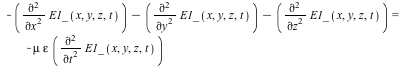PDEtools:-dchange({x = r*cos(theta), z = r*sin(theta)}, -(diff(diff(E1_(x, y, z, t), x), x))-(diff(diff(E1_(x, y, z, t), y), y))-(diff(diff(E1_(x, y, z, t), z), z)) = -mu*epsilon*(diff(diff(E1_(x, y, z, t), t), t)), simplify);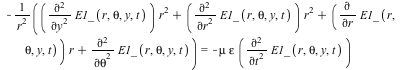subs(E1_(r, theta, y, t) = R(r)*exp(I*(-k*y+omega*t)), -((diff(diff(E1_(r, theta, y, t), y), y))*r^2+(diff(diff(E1_(r, theta, y, t), r), r))*r^2+(diff(E1_(r, theta, y, t), r))*r+diff(diff(E1_(r, theta, y, t), theta), theta))/r^2 = -mu*epsilon*(diff(diff(E1_(r, theta, y, t), t), t)));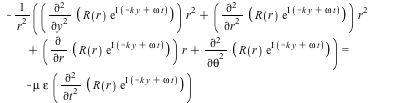dsolve((5));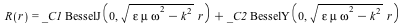So far this is all as expected.

Now I rerun this thing with Physics[Vectors] loaded rather than just Physics. Everything looks the same until I get to the line starting with subs(E1_ :

subs(E1_(r, theta, y, t) = R(r)*exp(I*(-k*y+omega*t)), -((diff(diff(E1_(r, theta, y, t), y), y))*r^2+(diff(diff(E1_(r, theta, y, t), r), r))*r^2+(diff(E1_(r, theta, y, t), r))*r+diff(diff(E1_(r, theta, y, t), theta), theta))/r^2 = -mu*epsilon*(diff(diff(E1_(r, theta, y, t), t), t)));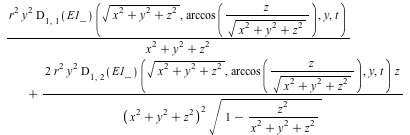plus many more lines of expressions. I am not sure what is going wrong; but after the dchange the code behaves funky: I have had simplify producing similarly weird expressions etc.

Note that on Maple 15 this all works as expected and no issues show up for me. If the version of Physics I have on Maple 17.02 is bad; how do I change that? (I have not tried to update Physics separately, and it isn't obvious to me where to find it for Maple 17). It is sort-of an issue as I want to use this as a demo for students, who in fact will be running Maple 18... but I am stuck at 17 (and often using 15 at home) so I need this to work there (17), too.

TIA,

Mac Dude

dchangeTest.mw﻿